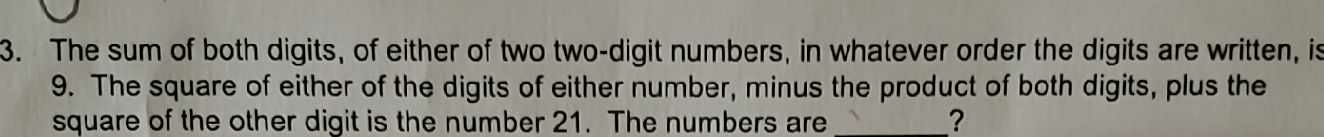### ¿Todavía tienes preguntas de matemáticas?

Pregunte a nuestros tutores expertos
Algebra
Pregunta3. The sum of both digits, of either of two two-digit numbers, in whatever order the digits are written, is 9. The square of either of the digits of either number, minus the product of both digits, plus the square of the other digit is the number $$21$$ . The numbers are